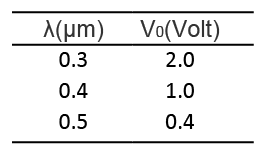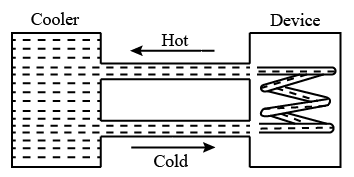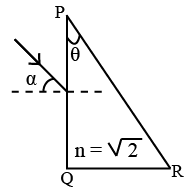Instructions

For the following questions answer them individually

Question 1

# In a historical experiment to determine Planck’s constant, a metal surface was irradiated with light of different wavelengths. The emitted photo electron energies were measured by applying a stopping potential. The relevant data for the wavelength ($$\lambda$$) of incident light and the corresponding stopping potential ($$V_0$$) are given below :Given that $$c = 3 \times 10^8 ms^{-1}$$ and $$e = 1.6 \times 10^{-19} C$$, Planck’s constant (in units of J s) found from such an experiment isQuestion 2

# A uniform wooden stick of mass 1.6 kg and length / rests in an inclined manneron a smooth, vertical wall of height A(</) such that a small portion of the stick extends beyond the wall. The reaction force of the wall on the stick is perpendicular to the stick. The stick makes an angle of $$30^\circ$$ with the wall and the bottom of the stick is on a roughfloor. The reaction of the wall on the stick is equal in magnitudeto the reaction of the floor on the stick. The ratio h/l and thefrictional force f at the bottom of the stick are (g = 10 ms$$^2$$)Question 3

# A water cooler of storage capacity 120 litres can cool water at a constant rate of P watts. In a closed circulation system (as shown schematically in the figure), the water from the cooler is used to cool an external device that generates constantly 3 kW of heat (thermal load). The temperature of water fed into the device cannot exceed $$30^\circ C$$ and the entire stored 120 litres of water is initially cooled to $$10^\circ C$$. The entire system is thermally insulated. The minimum value of P (in watts) for which the device can be operated for 3 hours is(Specific heat of water is 4.2 KJ kg$$^{-1}$$ K$$^{-1}$$ and the density of water is 1000 kg m$$^{-3}$$)Question 4

# A parallel beam of light is incident from air at an angle a on the side PQ of a right angled triangular prism of refractive index $$n = \sqrt{2}$$. Light undergoes total internal reflection in the prism at the face PR when a has a minimum value of $$45^\circ$$. The angle $$\theta$$ of the prism isQuestion 5

# An infinite line charge of uniform electric charge density $$\lambda$$ lies along the axis of an electrically conducting infinite cylindrical shell of radius R. At time t = 0, the space inside the cylinder is filled with a material of permittivity ε and electrical conductivity $$\sigma$$. The electrical conduction in the material follows Ohm’s law. Which one of the following graphs best describes the subsequent variation of the magnitude of current density j(t) at any point in the material?Question 6

# Highly excited states for hydrogen-like atoms (also called Rydberg states) with nuclear charge Ze are defined by their principal quantum number n, where n >> 1. Which of thefollowing statement(s) is(are) true?Question 7

# Two loudspeakers M and WN are located 20 m apart and emit sound at frequencies 118 Hz and 121 Hz, respectively. A caris initially at a point P , 1800 m away from the midpoint Qof the line MN and moves towards @ constantly at 60 km/hr along the perpendicular bisector of MN. It crosses Q and eventually reaches a point R, 1800 m away from Q. Let v(t) represent the beat frequency measured by a person sitting in the car at time t. Let $$V_P, V_Q$$ and $$V_R$$ be the beat frequencies measured at locations P, Q and R, respectively. The speed of sound in air is 330 ms$$^{-1}$$ Which of the following statement(s) is(are) true regarding the sound heard bythe person?Question 8

# An incandescent bulb has a thin filament of tungsten that is heated to high temperature by passing an electric current. The hot filament emits black-body radiation. The filament is observed to break up at randomlocations after a sufficiently long time of operation due to non-uniform evaporation of tungsten from the filament. If the bulb is powered at constant voltage, which of the following statement(s) is(are) true?Question 9

# A plano-convex lens is made ofa material of refractive index n. When a smallobject is placed 30 cm awayin front of the curved surface of the lens, an image of double the size of the object is produced. Dueto reflection from the convex surface of the lens, another faint image is observed at a distance of 10 cm away from the lens. Which of the following statement(s)is(are) true?Question 10

# A length-scale (J) depends on the permittivity ($$ε$$) of a dielectric material, Boltzmann constant ($$k_B$$), the absolute temperature (T), the number per unit volume (n) of certain charged particles, and the charge (q) carried by each of the particles. Which of the following expression(s) for l is(are) dimensionally correct?OR